# The ACECLUS Procedure

### Background

It is well known from the literature on nonparametric statistics that variances and, hence, covariances can be computed from pairwise differences instead of deviations from means. (For example, Puri and Sen (1971, pp. 51–52) show that the variance is a U statistic of degree 2.) Let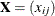be the data matrix with n observations (rows) and v variables (columns), and let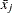be the mean of the jth variable. The sample covariance matrix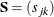is usually defined as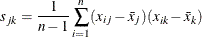The matrix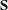can also be computed as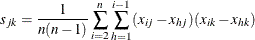Let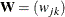be the pooled within-cluster covariance matrix, q be the number of clusters,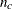be the number of observations in the cth cluster, and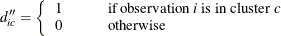The matrix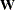is normally defined as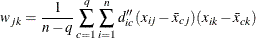where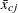is the mean of the jth variable in cluster c. Let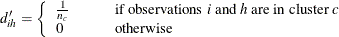The matrixcan also be computed as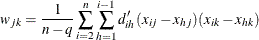If the clusters are not known,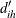cannot be determined. However, an approximation tocan be obtained by using instead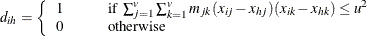where u is an appropriately chosen value and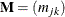is an appropriate metric. Let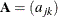be defined as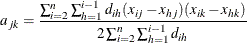If all of the following conditions hold,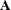equals:

• All within-cluster distances in the metric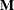are less than or equal to u.

• All between-cluster distances in the metricare greater than u.

• All clusters have the same number of members.

If the clusters are of unequal size,gives more weight to large clusters thandoes, but this discrepancy should be of little importance if the population within-cluster covariance matrices are equal. There might be large differences betweenandif the cutoff u does not discriminate between pairs in the same cluster and pairs in different clusters. Lack of discrimination might occur for one of the following reasons:

• The clusters are not well separated.

• The metricor the cutoff u is not chosen appropriately.

In the former case, little can be done to remedy the problem. The remaining question concerns how to chooseand u. Considerfirst. The best choice foris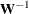, butis not known. The solution is to use an iterative algorithm:

1. Obtain an initial estimate of, such as the identity or the total-sample covariance matrix. See the INITIAL= option in the PROC ACECLUS statement for more information.

2. Letequal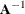.

3. Recomputeby using the preceding formula.

4. Repeat steps 2 and 3 until the estimate stabilizes.

Convergence is assessed by comparing values ofon successive iterations. Let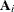be the value ofon the ith iteration and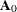be the initial estimate of. Let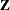be a user-specifiedmatrix. See the METRIC= option in the PROC ACECLUS statement for more information. The convergence measure is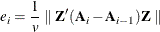where
indicates the Euclidean norm—that is, the square root of the sum of the squares of the elements of the matrix. In PROC ACECLUS,can be the identity or an inverse factor ofor diag(). Iteration stops when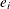falls below a user-specified value. See the CONVERGE= option or the MAXITER= option in the PROC ACECLUS statement for more information.

The remaining question of how to choose u has no simple answer. In practice, you must try several different values. PROC ACECLUS provides four different ways of specifying u:

• You can specify a constant value for u. This method is useful if the initial estimate ofis quite good. See the ABSOLUTE option and the THRESHOLD= option in the PROC ACECLUS statement for more information.

• You can specify a threshold value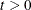that is multiplied by the root mean square distance between observations in the current metric on each iteration to give u. Thus, the value of u changes from iteration to iteration. This method is appropriate if the initial estimate ofis poor. See the THRESHOLD= option in the PROC ACECLUS statement for more information.

• You can specify a value p,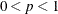, to be transformed into a distance u such that approximately a proportion p of the pairwise Mahalanobis distances between observations in a random sample from a multivariate normal distribution will be less than u in repeated sampling. The transformation can be computed only if the number of observations exceeds the number of variables, preferably by at least 10 percent. This method also requires a good initial estimate of. See the PROPORTION= option and the ABSOLUTE option in the PROC ACECLUS statement for more information.

• You can specify a value p,, to be transformed into a value t that is then multiplied by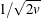times the root mean square distance between observations in the current metric on each iteration to yield u. The value of u changes from iteration to iteration. This method can be used with a poor initial estimate of. See the PROPORTION= option in the PROC ACECLUS statement for more information.

In most cases, the analysis should begin with the last method, using values of p between 0.5 and 0.01 and using the full covariance matrix as the initial estimate of.

Proportions p are transformed to distances t by using the formula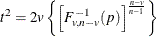where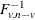is the quantile (inverse cumulative distribution) function of an F random variable with v anddegrees of freedom. The squared Mahalanobis distance between a single pair of observations sampled from a multivariate normal distribution is distributed astimes an F random variable with v anddegrees of freedom. The distances between two pairs of observations are correlated if the pairs have an observation in common. The quantile function is raised to the power given in the preceding formula to compensate approximately for the correlations among distances between pairs of observations that share a member. Monte Carlo studies indicate that the approximation is acceptable if the number of observations exceeds the number of variables by at least 10 percent.

Ifbecomes singular, step 2 in the iterative algorithm cannot be performed becausecannot be inverted. In this case, letbe the matrix as defined in discussing the convergence measure, and let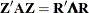, where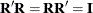and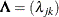is diagonal. Let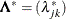be a diagonal matrix, where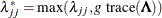, and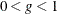is a user-specified singularity criterion (see the SINGULAR= option in the PROC ACECLUS statement for more information). Thenis computed as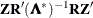.

The ACECLUS procedure differs from the method used by Art, Gnanadesikan, and Kettenring (1982) in several respects:

• The Art, Gnanadesikan, and Kettenring method uses the identity matrix as the initial estimate, whereas the ACECLUS procedure enables you to specify any symmetric matrix as the initial estimate and defaults to the total-sample covariance matrix. The default initial estimate in PROC ACECLUS is chosen to yield invariance under nonsingular linear transformations of the data but might sometimes obscure clusters that become apparent if the identity matrix is used.

• The Art, Gnanadesikan, and Kettenring method carries out all computations with SSCP matrices, whereas the ACECLUS procedure uses estimated covariance matrices because covariances are easier to interpret than crossproducts.

• The Art, Gnanadesikan, and Kettenring method uses the m pairs with the smallest distances to form the new estimate at each iteration, where m is specified by the user, whereas the ACECLUS procedure uses all pairs closer than a given cutoff value. Kettenring (1984) says that the m-closest-pairs method seems to give the user more direct control. PROC ACECLUS uses a distance cutoff because it yields a slight decrease in computer time and because in some cases, such as widely separated spherical clusters, the results are less sensitive to the choice of distance cutoff than to the choice of m. Much research remains to be done on this issue.

• The Art, Gnanadesikan, and Kettenring method uses a different convergence measure. Letbe computed on each iteration by using the m-closest-pairs method, and let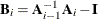, whereis the identity matrix. The convergence measure is equivalent to trace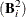.

Analyses of the Fisher (1936) iris data, consisting of measurements of petal and sepal length and width for 50 specimens from each of three iris species, are summarized in Table 23.1. The number of misclassified observations out of 150 is given for four clustering methods:

• k-means as implemented in PROC FASTCLUS with MAXC=3, MAXITER=99, and CONV=0

• Ward’s minimum variance method as implemented in PROC CLUSTER

• average linkage on Euclidean distances as implemented in PROC CLUSTER

• the centroid method as implemented in PROC CLUSTER

Each hierarchical analysis is followed by the TREE procedure with NCL=3 to determine cluster assignments at the three-cluster level. Clusters with 20 or fewer observations are discarded by using the DOCK=20 option. The observations in a discarded cluster are considered unclassified.

Each method is applied to the following data:

• the raw data

• the data standardized to unit variance by the STANDARD procedure

• two standardized principal components accounting for 95 percent of the standardized variance and having an identity total-sample covariance matrix, computed by the PRINCOMP procedure with the STD option

• four standardized principal components having an identity total-sample covariance matrix, computed by PROC PRINCOMP with the STD option

• the data transformed by PROC ACECLUS, using seven different settings of the PROPORTION= (P=) option

• four canonical variables having an identity pooled within-species covariance matrix, computed using the CANDISC procedure

Theoretically, the best results should be obtained by using the canonical variables from PROC CANDISC. PROC ACECLUS yields results comparable to those from PROC CANDISC for values of the PROPORTION= option ranging from 0.005 to 0.02. At PROPORTION=0.04, average linkage and the centroid method show some deterioration, but k-means and Ward’s method continue to produce excellent classifications. At larger values of the PROPORTION= option, all methods perform poorly, although no worse than with four standardized principal components.

Table 23.1: Number of Misclassified and Unclassified Observations Using the Fisher (1936) Iris Data

Clustering Method

Data

k-means

Ward’s

Centroid

Raw Data

16

16

25+12

14

Standardized Data

25

26

33+4

33+4

Two Standardized Principal Components

29

31

30+9

27+32

Four Standardized Principal Components

39

27

32+7

45+11

Transformed by ACECLUS, P=0.32

39

10+9

7+25

Transformed by ACECLUS, P=0.16

39

18+9

7+19

7+26

Transformed by ACECLUS, P=0.08

19

9

3+13

5+16

Transformed by ACECLUS, P=0.04

4

5

1+19

3+12

Transformed by ACECLUS, P=0.02

4

3

3

3

Transformed by ACECLUS, P=0.01

4

4

3

4

Transformed by ACECLUS, P=0.005

4

4

4

4

Canonical Variables

3

5

4

4+1

— A single number represents misclassified observations with no unclassified observations.

— Where two numbers are separated by a plus sign, the first is the number of observations;

the second is the number of unclassified observations.

This example demonstrates the following:

• PROC ACECLUS can produce results as good as those from the optimal transformation.

• PROC ACECLUS can be useful even when the within-cluster covariance matrices are moderately heterogeneous.

• The choice of the distance cutoff as specified by the PROPORTION= or the THRESHOLD= option is important, and several values should be tried.

• Commonly used transformations such as standardization and principal components can produce poor classifications.

Although experience with the Art, Gnanadesikan, and Kettenring and PROC ACECLUS methods is limited, the results so far suggest that these methods help considerably more often than they hinder the subsequent cluster analysis, especially with normal-mixture techniques such as k-means and Ward’s minimum variance method.